Examples

Chapter 2 Class 11 Relations and Functions
Serial order wise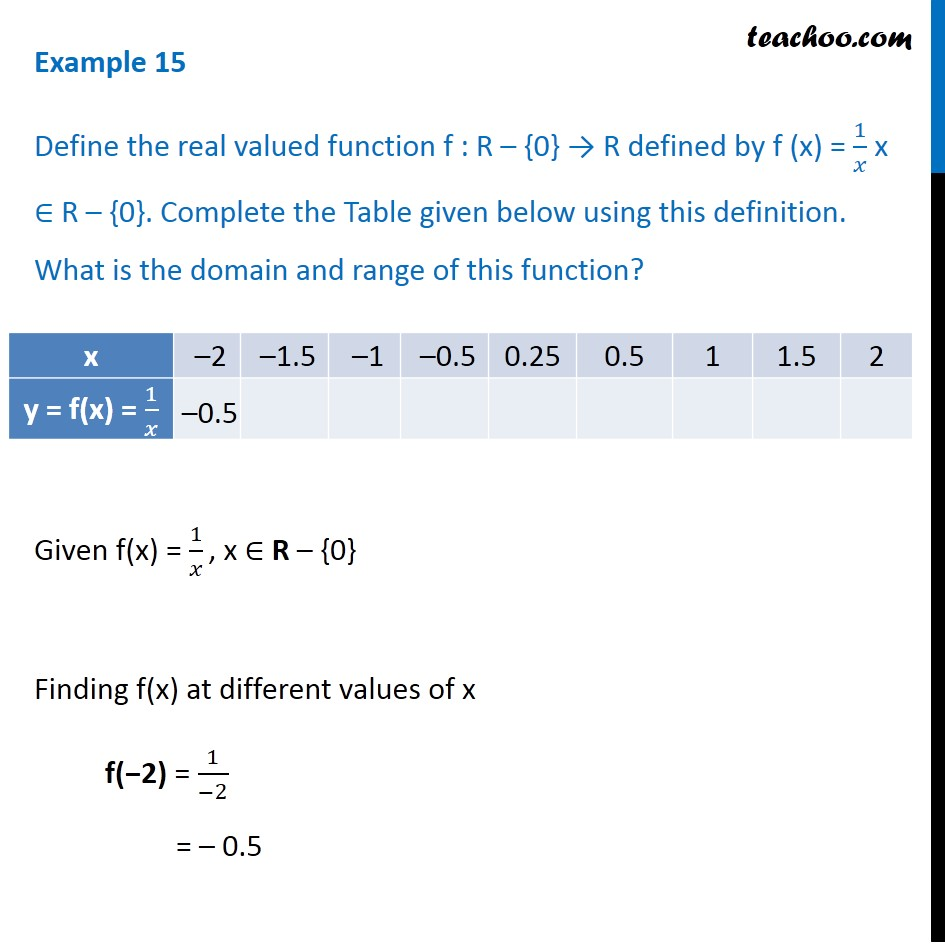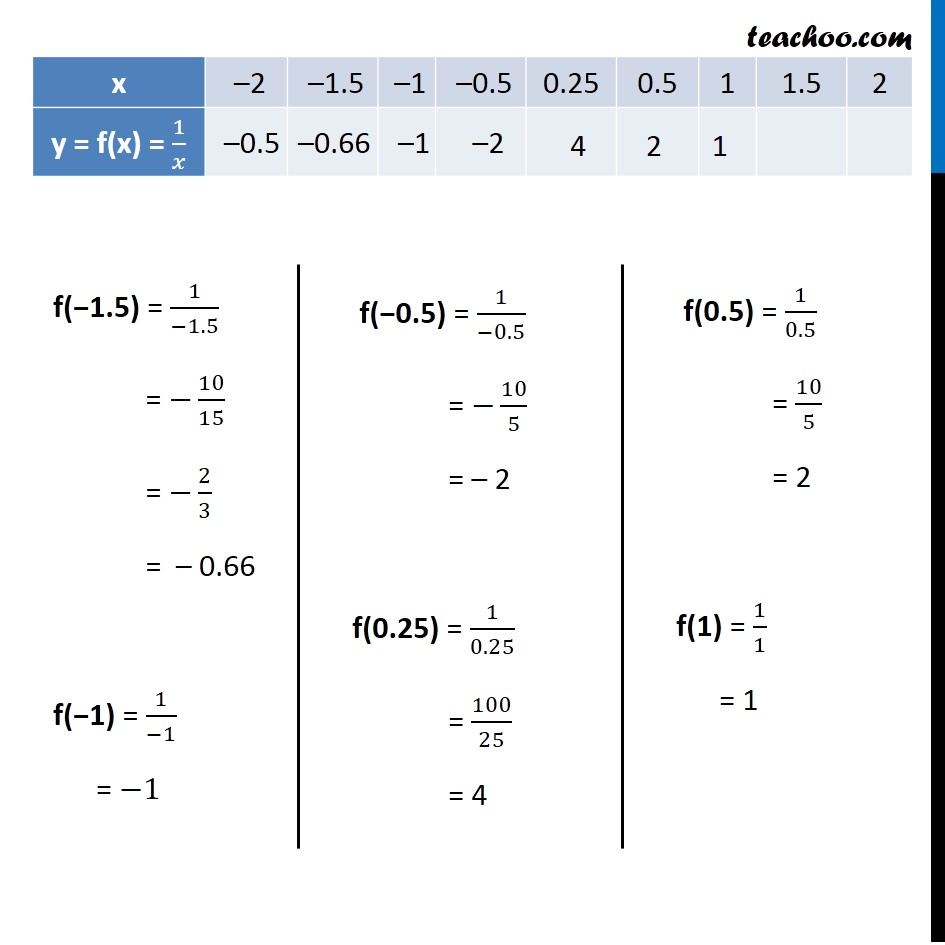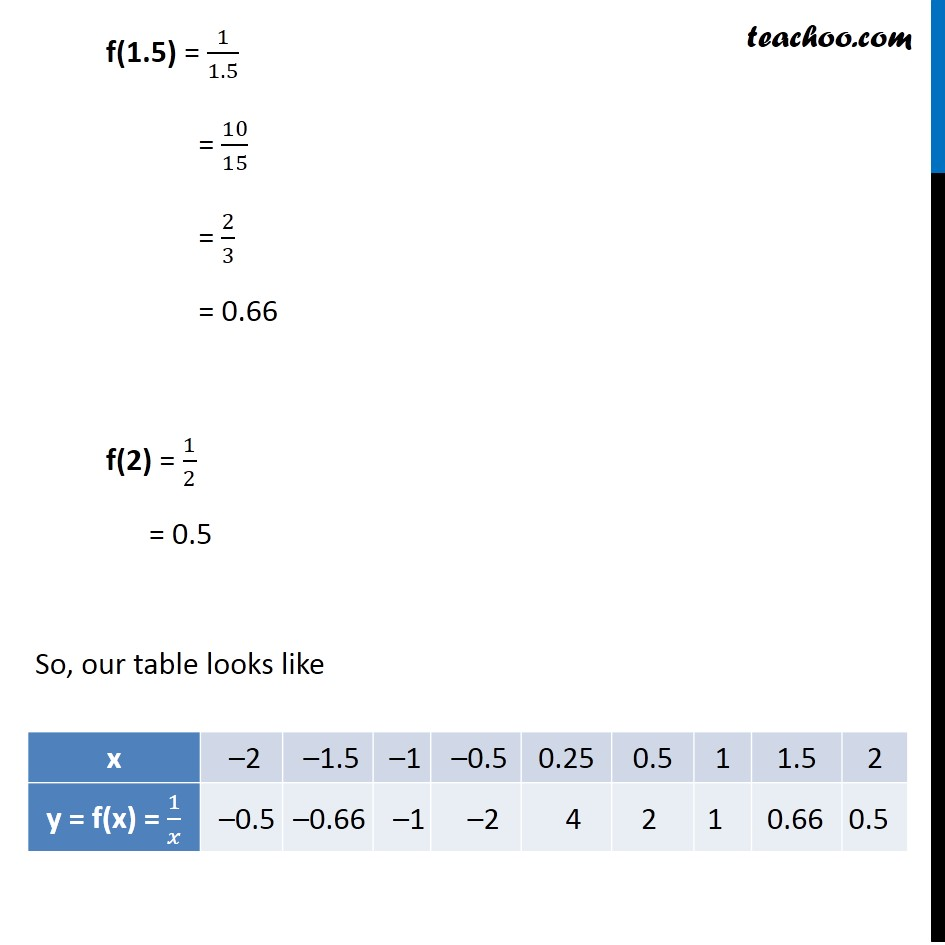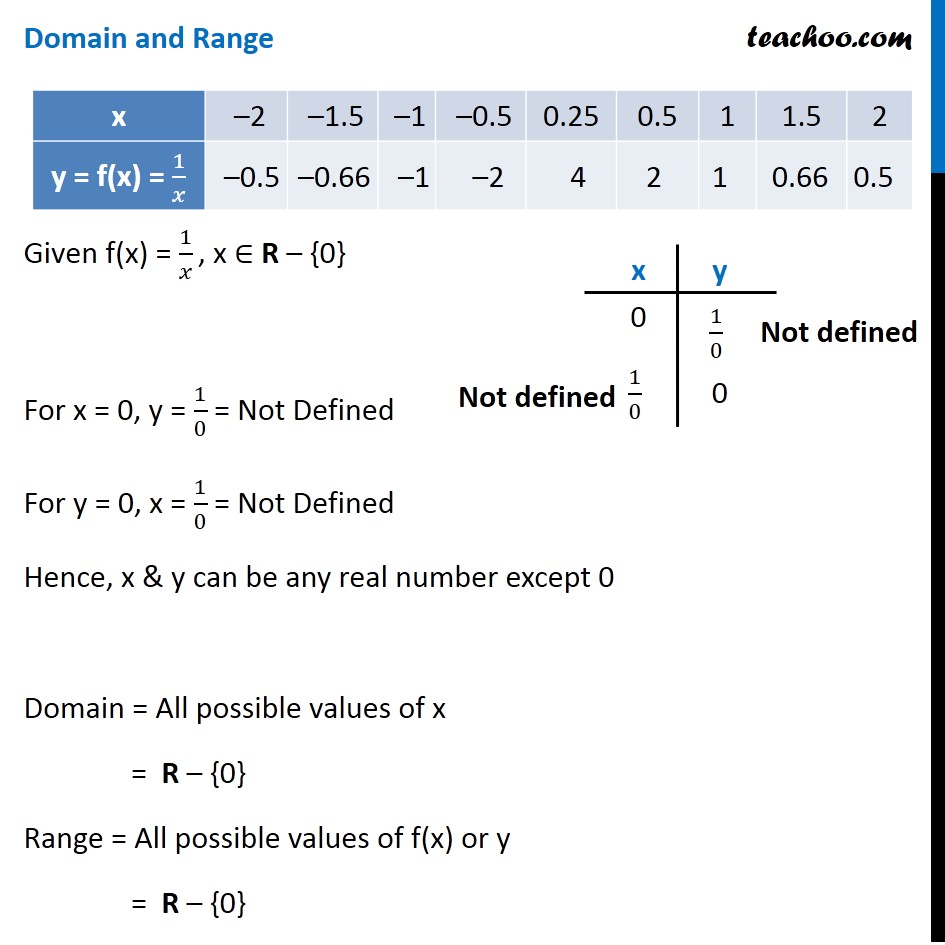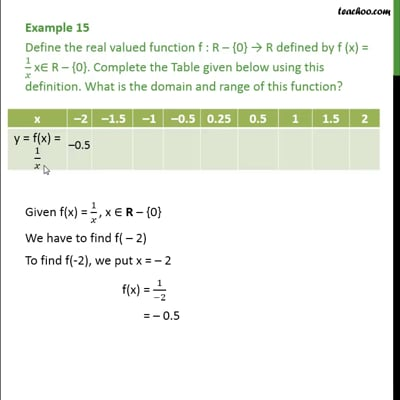This video is only available for Teachoo black users

Maths Crash Course - Live lectures + all videos + Real time Doubt solving!

### Transcript

Example 15 Define the real valued function f : R – {0} → R defined by f (x) = 1/𝑥 x ∈ R – {0}. Complete the Table given below using this definition. What is the domain and range of this function? Given f(x) = 1/𝑥 , x ∈ R – {0} Finding f(x) at different values of x f(−2) = 1/(−2) = – 0.5 f(−1.5) = 1/(−1.5) = −10/15 = −2/3 = – 0.66 f(−1) = 1/(−1) = −1 f(−0.5) = 1/(−0.5) = −10/5 = – 2 f(0.25) = 1/0.25 = 100/25 = 4 f(0.5) = 1/0.5 = 10/5 = 2 f(1) = 1/1 = 1 f(1.5) = 1/1.5 = 10/15 = 2/3 = 0.66 f(2) = 1/2 = 0.5 So, our table looks like Domain and Range Given f(x) = 1/𝑥 , x ∈ R – {0} For x = 0, y = 1/0 = Not Defined For y = 0, x = 1/0 = Not Defined Hence, x & y can be any real number except 0 Domain = All possible values of x = R – {0} Range = All possible values of f(x) or y = R – {0}